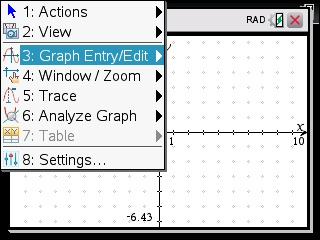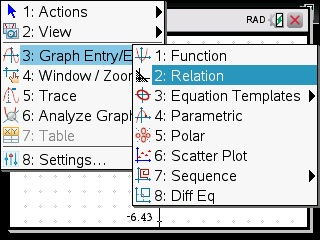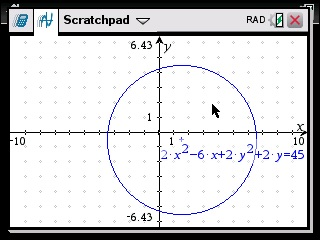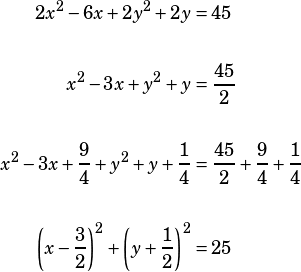Hi Mike,

Can you tell me how to do #27 (Section 4, Practice Test 6). I know how to do it by completing the square but was wondering if there is an easier way.

thanks!
Rachel

If you have a calculator that can graph the given equation without rearranging it (like an Nspire) then the easiest way is just to do that.If you don’t have one of those, though, then completing the square is 100% the way to go.Of course, since the formula for a circle is, that 25 on the right side of the equation above tells you the radius is 5.